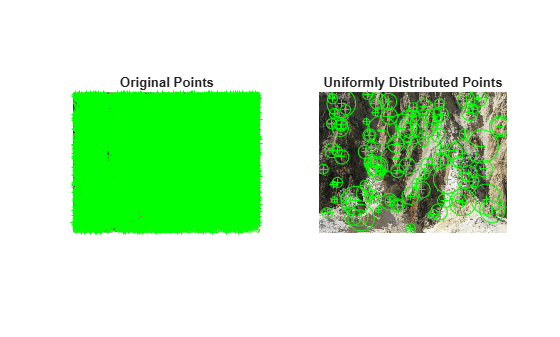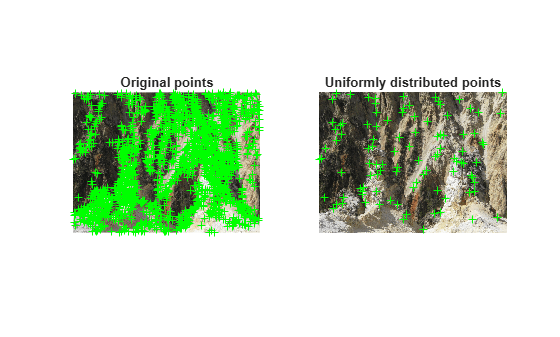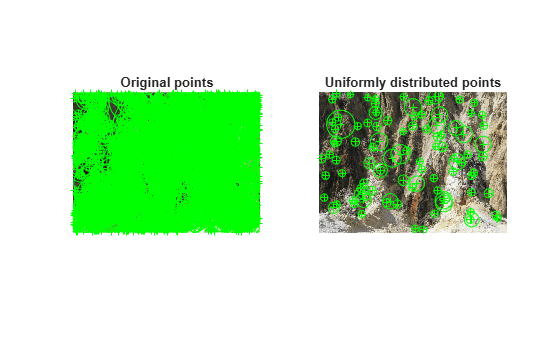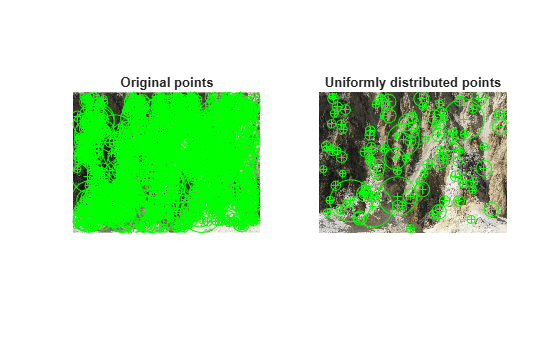# selectUniform

Select uniformly distributed subset of point features

## Syntax

``pointsOut = selectUniform(points,N,imageSize)``

## Description

example

````pointsOut = selectUniform(points,N,imageSize)` returns `N` uniformly distributed points with the strongest metrics.```

## Examples

collapse all

`im = imread("yellowstone_left.png");`

Detect and display KAZE features.

`points1 = detectKAZEFeatures(im2gray(im))`
```points1 = 6712x1 KAZEPoints array with properties: Location: [6712x2 single] Metric: [6712x1 single] Count: 6712 Scale: [6712x1 single] Orientation: [6712x1 single] ```
```subplot(1,2,1) imshow(im) hold on plot(points1) hold off title("Original Points")```

Select a uniformly distributed subset of points.

```numPoints = 100; points2 = selectUniform(points1,numPoints,size(im))```
```points2 = 100x1 KAZEPoints array with properties: Location: [100x2 single] Metric: [100x1 single] Count: 100 Scale: [100x1 single] Orientation: [100x1 single] ```
```subplot(1,2,2) imshow(im) hold on plot(points2) hold off title("Uniformly Distributed Points")````im = imread("yellowstone_left.png");`

Detect many corners by reducing the quality threshold.

`points1 = detectHarrisFeatures(im2gray(im),MinQuality=0.05);`

Plot image with detected corners.

```subplot(1,2,1); imshow(im); hold on plot(points1); hold off title("Original points");```

Select a uniformly distributed subset of points.

```numPoints = 100; points2 = selectUniform(points1,numPoints,size(im));```

Plot images showing original and subset of points.

```subplot(1, 2, 2); imshow(im); hold on plot(points2); hold off title("Uniformly distributed points");```Load an image into the workspace.

`im = imread("yellowstone_left.png");`

Detect many corners by reducing the quality threshold.

`points1 = detectBRISKFeatures(im2gray(im),MinQuality=0.05);`

Plot image with detected corners.

```subplot(1,2,1); imshow(im); hold on plot(points1); hold off title("Original points");```

Select a uniformly distributed subset of points.

```numPoints = 100; points2 = selectUniform(points1,numPoints,size(im));```

Plot images showing original and subset of points.

```subplot(1, 2, 2); imshow(im); hold on plot(points2); hold off title("Uniformly distributed points");````im = imread("yellowstone_left.png");`

Detect and display SURF features.

```points1 = detectSURFFeatures(im2gray(im)); subplot(1,2,1); imshow(im); hold on plot(points1); hold off title("Original points");```

Select a uniformly distributed subset of points.

```numPoints = 100; points2 = selectUniform(points1,numPoints,size(im));```

Plot images showing original and subset of points.

```subplot(1, 2, 2); imshow(im); hold on plot(points2); hold off title("Uniformly distributed points");```## Input Arguments

collapse all

Points object,specified as an M-by-2 matrix of M number of [x y] coordinates, or as one of the point feature objects described in Point Feature Types. The object contains information about the point features detected in the input image. To obtain points, use the appropriate detect function that pairs with the point feature type.

Number of uniformly distributed points to select, specified as an integer.

Size of image, specified as a 2-element vector for grayscale images or a 3-element vector for truecolor images.

## Output Arguments

collapse all

Uniformly distributed points, returned as a points feature object.

## Version History

Introduced in R2012a